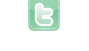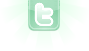## Problem with receiving damage and therefore removing the hearts.

Hello. This is a problem that I had for a long time now and I can't seem to solve this one after multiple hours. I have a health int in "Link" and multiple heart classes which are being created in "MyWorld". The Octorock (An Enemy) should when hitting / touching Link lower the health by 1 point and remove a heart. Same goes with the Projectile class which is being shot by the Octorock it should also (when Link is hit) remove his health by 1 and remove 1 of his hearts. With that problem I can't start up my "isGameOver" / setting it to true since my health never lowers. I'll post the codes in here one by one. This problem is ratherlarge complicated so I'd love to have some help! This first one is setting the heart classes into my world. (the heartclasses are just empty (Heart1.class / Heart2.class)
```public class MyWorld extends World
{
public int abstand = 120;
GreenfootSound OverWorld = new GreenfootSound("Overworld.mp3");

/**
* Constructor for objects of class MyWorld.
*
*/
public MyWorld()
{
// Create a new world with 800x600 cells with a cell size of 1x1 pixels.
super(800, 600, 1);

setBackground(new GreenfootImage("background.png"));

Octorock Octo = new Octorock();

Octorock Octo2 = new Octorock();

Octorock Octo3 = new Octorock();

Heart5 Heart = new Heart5();

Heart1 Heart1 = new Heart1();

Heart2 Heart2 = new Heart2();

Heart3 Heart3 = new Heart3();

Heart4 Heart4 = new Heart4();
for(int i=1;i<6;i++){
Wall Wall_i = new Wall();
addObject (Wall_i,737 - abstand, 537 - abstand);
}

Wall Wall_i = new Wall();

//Bar UIBar = new Bar();

Life Life = new Life();

OverWorld.play();
OverWorld.setVolume(25);

}

}
```
```public class Link extends Actor
{
/**
* Act - do whatever Link wants to do. This method is called whenever
* the 'Act' or 'Run' button gets pressed in the environment.
*/

// Pictures for Links movement and sword attack.
private GreenfootImage Down0 = new GreenfootImage("linkDown0.png");
private GreenfootImage Down1 = new GreenfootImage("linkDown1.png");
private GreenfootImage Left0 = new GreenfootImage("linkLeft0.png");
private GreenfootImage Left1 = new GreenfootImage("linkLeft1.png");
private GreenfootImage Right0 = new GreenfootImage("linkRight0.png");
private GreenfootImage Right1 = new GreenfootImage("linkRight1.png");
private GreenfootImage Up0 = new GreenfootImage("linkUp0.png");
private GreenfootImage Up1 = new GreenfootImage("linkUp1.png");
private GreenfootImage swordDown = new GreenfootImage("linkSwordDown.png");
private GreenfootImage swordLeft = new GreenfootImage("linkSwordLeft.png");
private GreenfootImage swordUp = new GreenfootImage("linkSwordUp.png");
private GreenfootImage swordRight = new GreenfootImage("linkSwordRight.png");

private GreenfootSound Sword = new GreenfootSound("LOZ_Sword.wav");
private GreenfootSound Die = new GreenfootSound("LOZ_Die.wav");

public int health = 5;

//Variable for Animation
private static int spin = 4;

public static int dmg = 1;

private boolean pressed = false; //checks if attack button got pressed

public static boolean isAttacking = false;
public static boolean isGameOver = false;
public static boolean isKnockback = false;

public void act()
{
keyMove();
attack();
wall();

//Checks if Link has ran out of hearts --> Leading to GameOver
if(isGameOver == true){
((MyWorld)getWorld()).OverWorld.stop();
Die.play();
Die.setVolume(75);
Greenfoot.delay(spin);
Greenfoot.delay(spin);
Greenfoot.delay(spin);
Greenfoot.delay(spin);
Greenfoot.delay(spin);
Greenfoot.delay(spin);
Greenfoot.delay(spin);
Greenfoot.delay(spin);
Greenfoot.delay(spin);
Greenfoot.delay(spin);
Greenfoot.delay(spin);
Greenfoot.delay(spin);
//move(Direction.DOWN, 500);
this.getImage().setTransparency(0);
Greenfoot.setWorld(new GameOverScreen());

}

}

public void keyMove()
{
if(Greenfoot.isKeyDown("Right")){
setLocation(getX()+5, getY());
if (getImage() == Right0) {
setImage (Right1);
}   else {
setImage(Right0);
}
}

if(Greenfoot.isKeyDown("Left")){
setLocation(getX()-5, getY());
if (getImage() == Left0) {
setImage (Left1);
}   else {
setImage(Left0);

}
}

if(Greenfoot.isKeyDown("Up")){
setLocation(getX(), getY()-5);
if (getImage() == Up0) {
setImage (Up1);
}   else {
setImage(Up0);
}
}

if(Greenfoot.isKeyDown("Down")){
setLocation(getX(), getY()+5);
if (getImage() == Down0) {
setImage (Down1);
}   else {
setImage(Down0);

}
}

}

public boolean canSee(Class clss)
{
Actor actor = getOneObjectAtOffset(0, 0, clss);
return actor != null;
}

public void wall() {
if(canSee(Wall.class) || canSee(Tree.class)) {
if(getImage() == Right0 || getImage() == Right1 || getImage() == swordRight) {
setLocation(getX() - 5 , getY());
}
if(getImage() == Left0 || getImage() == Left1 || getImage() == swordLeft) {
setLocation(getX() + 5, getY());
}
if(getImage() == Up0 || getImage() == Up1 || getImage() == swordUp) {
setLocation(getX() , getY() + 5);
}
if(getImage() == Down0 || getImage() == Down1 || getImage() == swordDown) {
setLocation(getX(), getY() - 5);
}
}
}

public void attack() {
if(Greenfoot.isKeyDown ("space")){
if(!pressed) {
if (getImage() == Right0 || getImage() == Right1) {
setImage(swordRight);
}
else {
if (getImage() == Left0 || getImage() == Left1) {
setImage (swordLeft);
}
if (getImage() == Up0 || getImage() == Up1) {
setImage (swordUp);
}
if (getImage() == Down0 || getImage() == Down1) {
setImage (swordDown);
}
}
Octorock enemy = (Octorock) getOneIntersectingObject(Octorock.class);
if (enemy != null)
{
enemy.takeDamage(dmg);
}
Sword.play();
Sword.setVolume(75);
pressed = true;

if (pressed = false) {
if (getImage() == swordUp) {
setImage (Up0);
}
if (getImage() == swordDown) {
setImage (Down0);
}
if (getImage() == swordLeft) {
setImage (Left0);
}
if (getImage() == swordRight) {
setImage (Right0);
}
}
}

}
}

public void takeDamage(int dmg) {
health -= dmg;
if (health < 1) {
isGameOver = true;
}
}

}   ```
This one is for the Octorock:
```public class Octorock extends Enemies
{
// Images
private GreenfootImage Down0 = new GreenfootImage("OctorockDown0.png");
private GreenfootImage Down1 = new GreenfootImage("OctorockDown1.png");
private GreenfootImage Left0 = new GreenfootImage("OctorockLeft0.png");
private GreenfootImage Left1 = new GreenfootImage("OctorockLeft1.png");
private GreenfootImage Right0 = new GreenfootImage("OctorockRight0.png");
private GreenfootImage Right1 = new GreenfootImage("OctorockRight1.png");
private GreenfootImage Up0 = new GreenfootImage("OctorockUp0.png");
private GreenfootImage Up1 = new GreenfootImage("OctorockUp1.png");

//Sound
private GreenfootSound Hit = new GreenfootSound("LOZ_Hit.wav");
private GreenfootSound Kill = new GreenfootSound("LOZ_Kill.wav");

//Variables
public static int currentDir = 0;
public static int health = 2;
public static int dmg = 1;
public static Projectile p = new Projectile();

/**
* Act - do whatever the Octorock wants to do. This method is called whenever
* the 'Act' or 'Run' button gets pressed in the environment.
*/
public void act()
{
moveOctorock();
wall();

}

public void shoot(int dir){
int x=-1,y=-1;
if(dir == 0){//right
x = this.getX()+20;
y = this.getY();
}
else if(dir==1){//down
x = this.getX();
y = this.getY()+20;
}
else if(dir==2){//left
x = this.getX()-20;
y = this.getY();
}
else if(dir==3){//up
x = this.getX();
y = this.getY()-20;
}
else if(x == -1 || y ==-1){
System.out.println("shooting error");
}
p.setRotation(getRotation());

p.resetShoot(dir);
}

/**
* moveOctorock - Moves Octorock randomly
*/
public void moveOctorock(){

Random generator = new Random(); //random number generator
int ranNum = generator.nextInt(250); //gets random number from 0-249
//random shoot
if(ranNum >200 && ranNum < 203 && !inKnockback){
shoot(direction);
}
else{
//when ranNum 0 rotates Octorock 90 degrees clockwise
if(ranNum == 0){
turn(90);
direction=direction+1;
imgRot = imgRot + 90;
if(direction == 4){ //allows direction to be 0,1,2, or 3 then resets to 0
direction =0;
imgRot = 0;
}
}
//when ranNum is 1 rotates Octorock 90 degrees counterclockwise
else if(ranNum == 1){
turn(-90);
direction=direction-1;
imgRot = imgRot - 90;
if(direction == -1){ //allows direction to be 0,1,2 or 3 then resets to 3
direction = 3;
imgRot = 270;
}
}
//when ranNum is >2 moves Octorock according to current direction
else if(ranNum >= 2){
//Move Octorock down if he won't hit border

if(direction == 1 && (((MyWorld) getWorld()).getHeight()-getY())>10){
this.getImage().rotate(270); //rotates image to be oriented correctly
move(2);
}
//Move Octorock left if he won't hit border
else if(direction == 2 && getX() > 10 ){
this.getImage().rotate(imgRot); //rotates image to be oriented correctly
move(2);
}
//Move Octorock up if he won't hit border
else if(direction == 3 && getY() > 60){
this.getImage().rotate(90); //roates image to be oriented correctly
move(2);
}
//Move Octorock right if he won't hit border
else if(direction == 0 && (((MyWorld) getWorld()).getWidth()-getX())>10){
this.getImage().rotate(imgRot); //rotates image to be oriented correctly
move(2);
}
//Rotate 90 degrees clockwise if current direction will hit border
else{
turn(90);
direction=direction+1;
currentDir=direction;
imgRot=imgRot+90;
if(direction == 4){
direction=0;
currentDir=0;
imgRot=0;
}
}

}//end if finished
else {
if(direction == 1 && (((MyWorld) getWorld()).getHeight()-getY())>10){
this.getImage().rotate(270); //rotates image to be oriented correctly
move(2);}

else if(direction == 2 && getX() > 10){
this.getImage().rotate(imgRot); //rotates image to be oriented correctly
move(2);
}

else if(direction == 3 && getY() > 60){
this.getImage().rotate(90); //roates image to be oriented correctly
move(2);
}
else if(direction == 0 && (((MyWorld) getWorld()).getWidth()-getX())>10){
this.getImage().rotate(imgRot); //rotates image to be oriented correctly
move(2);
}
else{
turn(90);
direction=direction+1;
imgRot=imgRot+90;
if(direction == 4){
direction=0;
imgRot=0;
}
}
}
if(direction == 1 && (((MyWorld) getWorld()).getHeight()-getY())>10){
this.getImage().rotate(270); //rotates image to be oriented correctly
move(2);}

else if(direction == 2 && getX() > 10){
this.getImage().rotate(imgRot); //rotates image to be oriented correctly
move(2);
}

else if(direction == 3 && getY() > 60){
this.getImage().rotate(90); //roates image to be oriented correctly
move(2);
}
else if(direction == 0 && (((MyWorld) getWorld()).getWidth()-getX())>10){
this.getImage().rotate(imgRot); //rotates image to be oriented correctly
move(2);
}
else{
turn(90);
direction=direction+1;
imgRot=imgRot+90;
if(direction == 4){
direction=0;
imgRot=0;
}
}
}

} //end moveOctorock()
public void wall() {
if(canSee(Wall.class) || canSee(Tree.class) || atWorldEdge() ==true) {
if(getImage() == Right0 || getImage() == Right1) {
setLocation(getX() - 5 , getY());
turn(180);
}
if(getImage() == Left0 || getImage() == Left1) {
setLocation(getX() + 5, getY());
turn(180);
}
if(getImage() == Up0 || getImage() == Up1) {
setLocation(getX() , getY() - 5);
turn(180);
}
if(getImage() == Down0 || getImage() == Down1) {
setLocation(getX(), getY() - 5);
turn(180);
}
}
}

public boolean canSee(Class clss)
{
Actor actor = getOneObjectAtOffset(0, 0, clss);
return actor != null;
}

public boolean atWorldEdge()
{
if(getX() < 20 || getX() > getWorld().getWidth() - 20)
return true;
if(getY() < 20 || getY() > getWorld().getHeight() - 20)
return true;
else
return false;
}

if (enemy != null)
{
enemy.takeDamage(dmg);
}

}

public void takeDamage(int dmg) {
health -= dmg;
Hit.play();
Hit.setVolume(75);
if (health < 1) {
getWorld().removeObject(this);
Kill.play();
Kill.setVolume(75);
}
}

}```
And last but not least the Projectile:
```public class Projectile extends UI
{
private int timer;

private static final int shootLength=100;
private static int currShoot=shootLength;
private int direction=0;
private int imgRot=0;
public boolean alive=true;
private boolean finish=false;
private boolean turn=false;

public static int dmg = 1;

/**
* Act - do whatever the Projectile wants to do. This method is called whenever
* the 'Act' or 'Run' button gets pressed in the environment.
*/
public void act()
{
removeSelf(); // removes Object after hitting Link, A Wall, or the Edge of the World.
shoot(); //moves the Projectile the way the Octorock is facing
removeAfterTime(); //removes Projectile after a while from the World

}

public void shoot(){
if(currShoot <= shootLength){
if(direction == 0 && !turn){
turn=true;
}
else if(direction ==1 && !turn){
imgRot=90;
turn(imgRot);
turn=true;
}
else if(direction ==2 && !turn){
imgRot = 180;
turn(imgRot);
turn=true;
}
else if(direction ==3 && !turn){
imgRot=-90;
turn(imgRot);
turn=true;
}

move(10);
currShoot++;
}

}

public void takeDamage(int dmg) {
if (enemy != null)
{
enemy.takeDamage(dmg);
}
}

/**
* removeSelf()
* remove projectile from the world
*/
public void removeSelf()
{
if(isTouching(Link.class) || isTouching(Wall.class) || atWorldEdge() ==true) {
this.getWorld().removeObject(this);
}
alive = false;
}
/**
* removeSelf()
* removes the Projectile after OnScreenTime
*/
public void removeAfterTime() {
if(++timer == 50) {
this.getWorld().removeObject(this);
return;
}
}

/**
* resetShoot()
* this method resets the variables which allow the animation of the shoot to start
* @param dir -  current direction the octorock is facing
*/
public void resetShoot(int dir){
if(currShoot < shootLength){ return;}
if(!finish){
direction = dir;
finish=true;
}
currShoot=0;
}

public boolean atWorldEdge()
{
if(getX() < 20 || getX() > getWorld().getWidth() - 20)
return true;
if(getY() < 20 || getY() > getWorld().getHeight() - 20)
return true;
else
return false;
}

}```
Okay that's that. I hope someone can help me out with it. I am quite new to all this Java / Greenfoot stuff so I'd appreciate if someone could help me.